# Removing Watched Server Objects

## Stop Watching

Enable Watching and Stop Watching on the targets within your server environment.

Note:  If you choose to Stop Watching a target, remember to reclaim the license for that target, allowing you to apply it elsewhere. For more information, see the License Management article.
Note:  You can use PowerShell to stop watching targets. See the PowerShell Module article for more information.
Note:  The Inventory node provides a read-only view of what you are watching. See the Inventory View article for details.

To Stop Watching a target in SQL Sentry, complete one of the following:

### Using the Navigator pane

Stop watching a target through the Navigator pane:

View > Navigator > Desired Target > Stop Watching

1. Open the Navigator pane (View > Navigator), and then select your desired target.
2. Right-click on the desired target, and then select Stop Watching.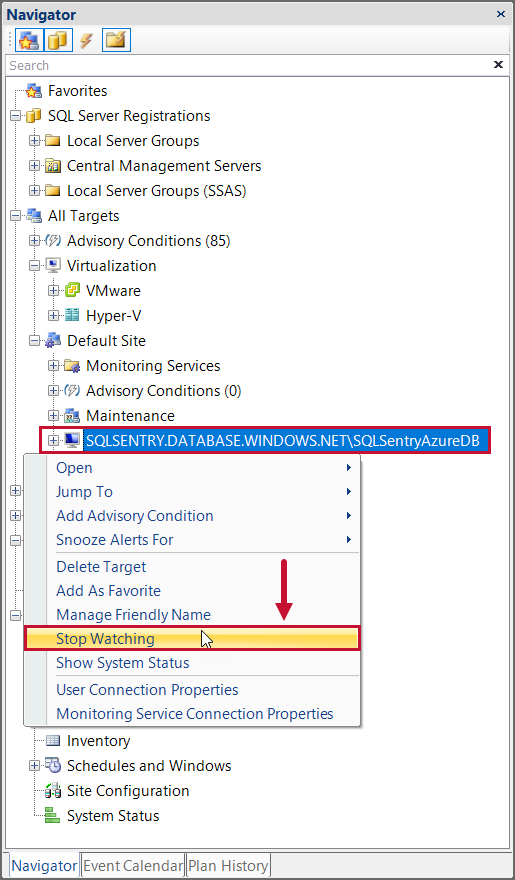Stop watching a target hosting a SQL Server instance through the Navigator pane:

View > Navigator > Desired Target > Desired Instance > Stop Watching Instance

1. Open the Navigator pane (View > Navigator), and then expand your desired target.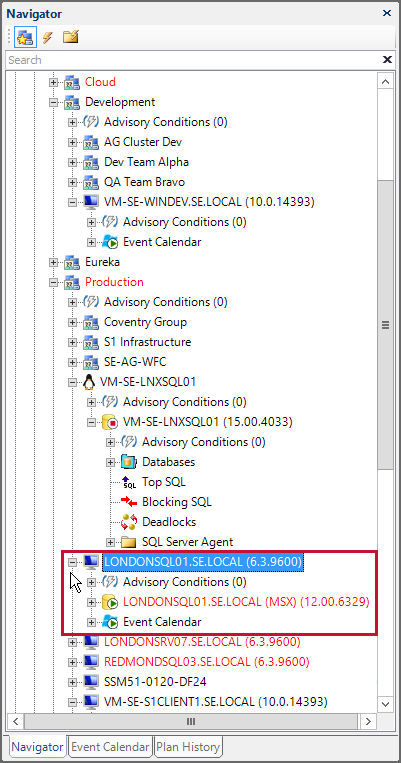2. Right click on the SQL Server Instance, and then select Stop Watching Instance.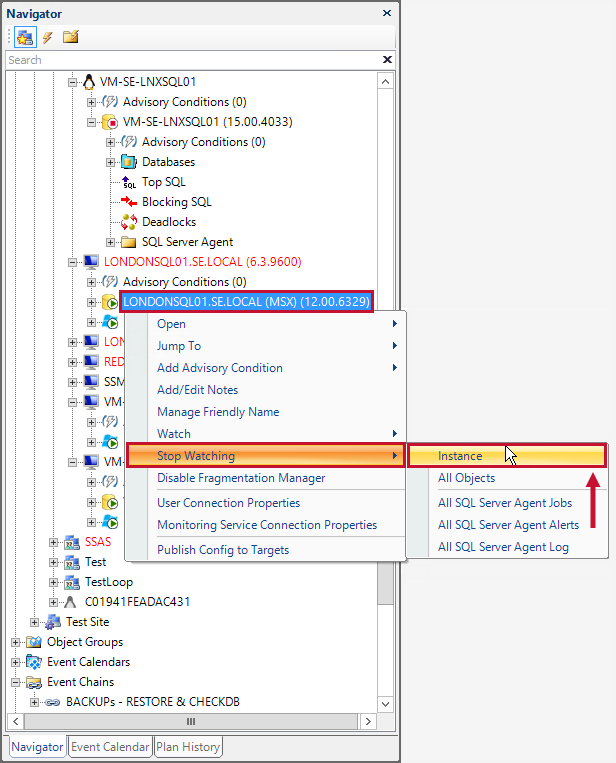3. Right click on the target, and then select Stop Watching

### Using Performance Overview

Stop watching through Performance Overview:

1. Right-click All Targets (View > Navigator > All Targets), select Open, and then select Performance Overview. This opens the All Targets tab.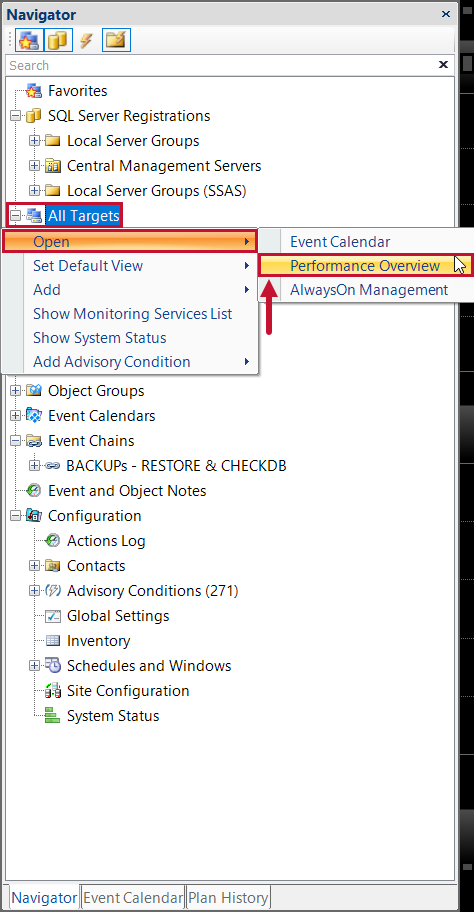2. Right-click on the desired target, then select Stop Watching.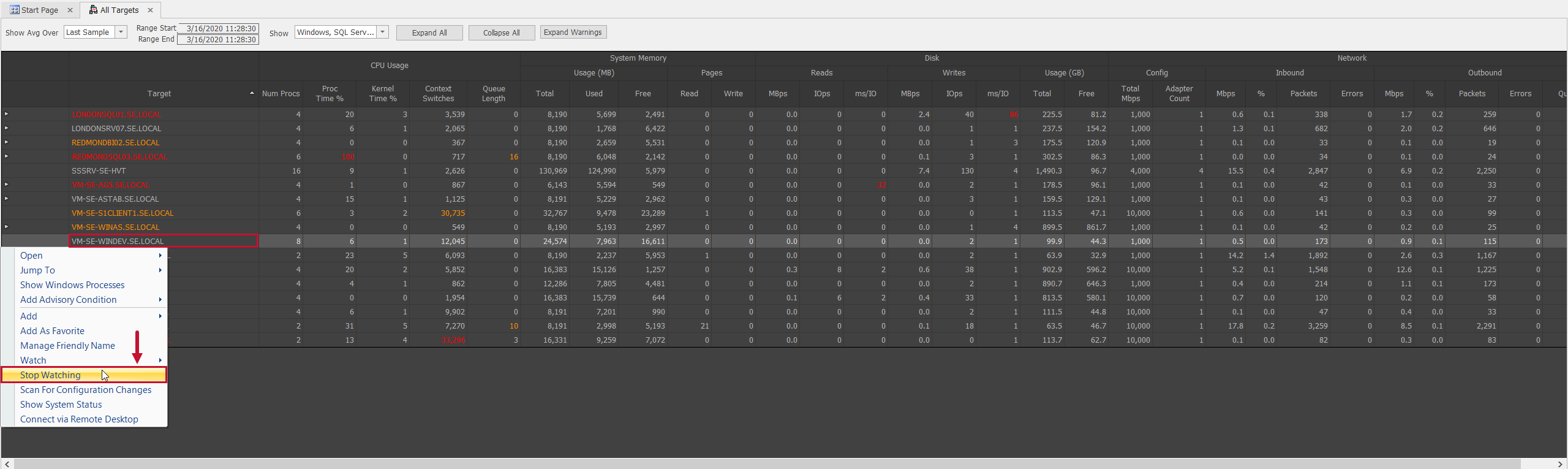## Removing Watched Server Objects

Note:  When you watch a SQL Server with Event Manager, SQL Sentry places a few objects in MSDB that facilitate its lightweight polling architecture. No agents are placed on the server. This enables SQL Sentry to monitor the server with a performance overhead that's typically less than SQL Agent. For more information about watched server objects, see the Watched Server Objects topic.

If you've stopped watching a SQL Server instance or Azure SQL Database target with SQL Sentry, and don't have plans to Watch it again, scripts are provided to automate the process of removing the objects SQL Sentry places on a watched target.

Important:  Stop Watching the server through the SQL Sentry client before attempting to remove the object.

### SQL Server 2000 Instances

 ```--SQL Server 2000 ```USE msdb if exists (select * from dbo.sysobjects where id = object_id(N'[dbo].[sp_sentry_mail]') and OBJECTPROPERTY(id, N'IsProcedure') = 1) drop procedure [dbo].[sp_sentry_mail] if exists (select * from dbo.sysobjects where id = object_id(N'[dbo].[sp_sentry_mail_20]') and OBJECTPROPERTY(id, N'IsProcedure') = 1) drop procedure [dbo].[sp_sentry_mail_20] if exists (select * from dbo.sysobjects where id = object_id(N'[dbo].[SQLSentryEmails_20]') and OBJECTPROPERTY(id, N'IsUserTable') = 1) drop table [dbo].[SQLSentryEmails_20] if exists (select * from dbo.sysobjects where id = object_id(N'[dbo].[spGetBlockInfo_20]') and OBJECTPROPERTY(id, N'IsProcedure') = 1) drop procedure [dbo].[spGetBlockInfo_20] if exists (select * from dbo.sysobjects where id = object_id(N'[dbo].[spGetBlockInfo_Pre8sp3]') and OBJECTPROPERTY(id, N'IsProcedure') = 1) drop procedure [dbo].[spGetBlockInfo_Pre8sp3] GO BEGIN TRANSACTION DECLARE @JobID BINARY(16) DECLARE @ReturnCode INT SELECT @ReturnCode = 0 -- Delete the job with the same name (if it exists) SELECT @JobID = job_id FROM msdb.dbo.sysjobs WHERE (name = N'SQL Sentry 2.0 Queue Monitor') IF (@JobID IS NOT NULL) BEGIN -- Check if the job is a multi-server job IF (EXISTS (SELECT * FROM msdb.dbo.sysjobservers WHERE (job_id = @JobID) AND (server_id <> 0))) BEGIN -- There is, so abort the script RAISERROR (N'Unable to import job ''SQL Sentry Queue Monitor'' since there is already a multi-server job with this name.', 16, 1) GOTO QuitWithRollback END ELSE -- Delete the [local] job EXECUTE msdb.dbo.sp_delete_job @job_name = N'SQL Sentry 2.0 Queue Monitor' SELECT @JobID = NULL END COMMIT TRANSACTION GOTO EndSave QuitWithRollback: IF (@@TRANCOUNT > 0) ROLLBACK TRANSACTION EndSave: GO if exists (select * from dbo.sysobjects where id = object_id(N'[dbo].[spGetJobInfo_20]') and OBJECTPROPERTY(id, N'IsProcedure') = 1) drop procedure [dbo].[spGetJobInfo_20] if exists (select * from dbo.sysobjects where id = object_id(N'[dbo].[spGetDTSLog_20]') and OBJECTPROPERTY(id, N'IsProcedure') = 1) drop procedure [dbo].[spGetDTSLog_20] if exists (select * from dbo.sysobjects where id = object_id(N'[dbo].[spQueueHeartbeat_20]') and OBJECTPROPERTY(id, N'IsProcedure') = 1) drop procedure [dbo].[spQueueHeartbeat_20] if exists (select * from dbo.sysobjects where id = object_id(N'[dbo].[spQueueJob_Start_20]') and OBJECTPROPERTY(id, N'IsProcedure') = 1) drop procedure [dbo].[spQueueJob_Start_20] if exists (select * from dbo.sysobjects where id = object_id(N'[dbo].[spQueueJob_End_20]') and OBJECTPROPERTY(id, N'IsProcedure') = 1) drop procedure [dbo].[spQueueJob_End_20] if exists (select * from dbo.sysobjects where id = object_id(N'[dbo].[spQueueMonitor_20]') and OBJECTPROPERTY(id, N'IsProcedure') = 1) drop procedure [dbo].[spQueueMonitor_20] if exists (select * from dbo.sysobjects where id = object_id(N'[dbo].[spReadLogFile_20]') and OBJECTPROPERTY(id, N'IsProcedure') = 1) drop procedure [dbo].[spReadLogFile_20] if exists (select * from dbo.sysobjects where id = object_id(N'[dbo].[SQLSentryQueueLog_20]') and OBJECTPROPERTY(id, N'IsUserTable') = 1) drop table [dbo].[SQLSentryQueueLog_20] if exists (select * from dbo.sysobjects where id = object_id(N'[dbo].[SQLSentryLogCache_20]') and OBJECTPROPERTY(id, N'IsUserTable') = 1) drop table [dbo].[SQLSentryLogCache_20] if exists (select * from dbo.sysobjects where id = object_id(N'[dbo].[SQLSentryLogCacheDTS_20]') and OBJECTPROPERTY(id, N'IsUserTable') = 1) drop table [dbo].[SQLSentryLogCacheDTS_20] GO BEGIN TRANSACTION DECLARE @JobID BINARY(16) DECLARE @ReturnCode INT SELECT @ReturnCode = 0 -- Delete the job with the same name (if it exists) SELECT @JobID = job_id FROM msdb.dbo.sysjobs WHERE (name = N'SQL Sentry 2.0 Alert Trap') IF (@JobID IS NOT NULL) BEGIN -- Check if the job is a multi-server job IF (EXISTS (SELECT * FROM msdb.dbo.sysjobservers WHERE (job_id = @JobID) AND (server_id <> 0))) BEGIN -- There is, so abort the script RAISERROR (N'Unable to import job ''SQL Sentry Alert Trap'' since there is already a multi-server job with this name.', 16, 1) GOTO QuitWithRollback END ELSE -- Delete the [local] job EXECUTE msdb.dbo.sp_delete_job @job_name = N'SQL Sentry 2.0 Alert Trap' SELECT @JobID = NULL END COMMIT TRANSACTION GOTO EndSave QuitWithRollback: IF (@@TRANCOUNT > 0) ROLLBACK TRANSACTION EndSave: GO if exists (select * from dbo.sysobjects where id = object_id(N'[dbo].[spTrapAlert_20]') and OBJECTPROPERTY(id, N'IsProcedure') = 1) drop procedure [dbo].[spTrapAlert_20] if exists (select * from dbo.sysobjects where id = object_id(N'[dbo].[spSetupAlertsTrap_20]') and OBJECTPROPERTY(id, N'IsProcedure') = 1) drop procedure [dbo].[spSetupAlertsTrap_20] if exists (select * from dbo.sysobjects where id = object_id(N'[dbo].[SQLSentryAlertLog_20]') and OBJECTPROPERTY(id, N'IsUserTable') = 1) drop table [dbo].[SQLSentryAlertLog_20] if exists (select * from dbo.sysobjects where id = object_id(N'[dbo].[SQLSentryLogData_20]') and OBJECTPROPERTY(id, N'IsUserTable') = 1) drop table [dbo].[SQLSentryLogData_20] if exists (select * from dbo.sysobjects where id = object_id(N'[dbo].[SQLSentryObjectVersion_20]') and OBJECTPROPERTY(id, N'IsUserTable') = 1) drop table [dbo].[SQLSentryObjectVersion_20] if exists (select * from dbo.sysobjects where id = object_id(N'[dbo].[fnGetSQL_20]') and OBJECTPROPERTY(id, N'IsScalarFunction') = 1) drop function [dbo].[fnGetSQL_20] if exists (select * from dbo.sysobjects where id = object_id(N'[dbo].[fnGetWaittypeDesc_20]') and OBJECTPROPERTY(id, N'IsScalarFunction') = 1) drop function [dbo].[fnGetWaittypeDesc_20]``````

### SQL Server 2005+ Instances

 ```--SQL Server 2005+ ```USE msdb if exists (select * from sys.objects where object_id = object_id(N'[dbo].[sp_sentry_mail]') and OBJECTPROPERTY(object_id, N'IsProcedure') = 1) drop procedure [dbo].[sp_sentry_mail] if exists (select * from sys.objects where object_id = object_id(N'[dbo].[sp_sentry_mail_20]') and OBJECTPROPERTY(object_id, N'IsProcedure') = 1) drop procedure [dbo].[sp_sentry_mail_20] if exists (select * from sys.objects where object_id = object_id(N'[dbo].[sp_sentry_dbmail_20]') and OBJECTPROPERTY(object_id, N'IsProcedure') = 1) drop procedure [dbo].[sp_sentry_dbmail_20] if exists (select * from sys.objects where object_id = object_id(N'[dbo].[SQLSentryEmails_20]') and OBJECTPROPERTY(object_id, N'IsUserTable') = 1) drop table [dbo].[SQLSentryEmails_20] if exists (select * from sys.objects where object_id = object_id(N'[dbo].[SQLSentryDBEmails_Attachments_20]') and OBJECTPROPERTY(object_id, N'IsUserTable') = 1) drop table [dbo].[SQLSentryDBEmails_Attachments_20] if exists (select * from sys.objects where object_id = object_id(N'[dbo].[SQLSentryDBEmails_20]') and OBJECTPROPERTY(object_id, N'IsUserTable') = 1) drop table [dbo].[SQLSentryDBEmails_20] if exists (select * from sys.objects where object_id = object_id(N'[dbo].[spGetBlockInfo_20]') and OBJECTPROPERTY(object_id, N'IsProcedure') = 1) drop procedure [dbo].[spGetBlockInfo_20] if exists (select * from sys.objects where object_id = object_id(N'[dbo].[spGetBlockInfo_Pre8sp3]') and OBJECTPROPERTY(object_id, N'IsProcedure') = 1) drop procedure [dbo].[spGetBlockInfo_Pre8sp3] if exists (select * from sys.objects where object_id = object_id(N'[dbo].[spGetQueryStatsData]') and OBJECTPROPERTY(object_id, N'IsProcedure') = 1) drop procedure [dbo].[spGetQueryStatsData] if exists (select * from sys.objects where object_id = object_id(N'[dbo].[spGetProcedureStatsData]') and OBJECTPROPERTY(object_id, N'IsProcedure') = 1) drop procedure [dbo].[spGetProcedureStatsData] GO BEGIN TRANSACTION DECLARE @JobID BINARY(16) DECLARE @ReturnCode INT SELECT @ReturnCode = 0 -- Delete the job with the same name (if it exists) SELECT @JobID = job_id FROM msdb.dbo.sysjobs WHERE (name = N'SQL Sentry 2.0 Queue Monitor') IF (@JobID IS NOT NULL) BEGIN -- Check if the job is a multi-server job IF (EXISTS (SELECT * FROM msdb.dbo.sysjobservers WHERE (job_id = @JobID) AND (server_id <> 0))) BEGIN -- There is, so abort the script RAISERROR (N'Unable to import job ''SQL Sentry Queue Monitor'' since there is already a multi-server job with this name.', 16, 1) GOTO QuitWithRollback END ELSE -- Delete the [local] job EXECUTE msdb.dbo.sp_delete_job @job_name = N'SQL Sentry 2.0 Queue Monitor' SELECT @JobID = NULL END COMMIT TRANSACTION GOTO EndSave QuitWithRollback: IF (@@TRANCOUNT > 0) ROLLBACK TRANSACTION EndSave: GO if exists (select * from sys.objects where object_id = object_id(N'[dbo].[spGetJobInfo_20]') and OBJECTPROPERTY(object_id, N'IsProcedure') = 1) drop procedure [dbo].[spGetJobInfo_20] if exists (select * from sys.objects where object_id = object_id(N'[dbo].[spGetDTSLog_20]') and OBJECTPROPERTY(object_id, N'IsProcedure') = 1) drop procedure [dbo].[spGetDTSLog_20] if exists (select * from sys.objects where object_id = object_id(N'[dbo].[spQueueHeartbeat_20]') and OBJECTPROPERTY(object_id, N'IsProcedure') = 1) drop procedure [dbo].[spQueueHeartbeat_20] if exists (select * from sys.objects where object_id = object_id(N'[dbo].[spQueueJob_Start_20]') and OBJECTPROPERTY(object_id, N'IsProcedure') = 1) drop procedure [dbo].[spQueueJob_Start_20] if exists (select * from sys.objects where object_id = object_id(N'[dbo].[spQueueJob_End_20]') and OBJECTPROPERTY(object_id, N'IsProcedure') = 1) drop procedure [dbo].[spQueueJob_End_20] if exists (select * from sys.objects where object_id = object_id(N'[dbo].[spQueueMonitor_20]') and OBJECTPROPERTY(object_id, N'IsProcedure') = 1) drop procedure [dbo].[spQueueMonitor_20] if exists (select * from sys.objects where object_id = object_id(N'[dbo].[spReadLogFile_20]') and OBJECTPROPERTY(object_id, N'IsProcedure') = 1) drop procedure [dbo].[spReadLogFile_20] if exists (select * from sys.objects where object_id = object_id(N'[dbo].[SQLSentryQueueLog_20]') and OBJECTPROPERTY(object_id, N'IsUserTable') = 1) drop table [dbo].[SQLSentryQueueLog_20] if exists (select * from sys.objects where object_id = object_id(N'[dbo].[SQLSentryLogCache_20]') and OBJECTPROPERTY(object_id, N'IsUserTable') = 1) drop table [dbo].[SQLSentryLogCache_20] if exists (select * from sys.objects where object_id = object_id(N'[dbo].[SQLSentryLogCacheDTS_20]') and OBJECTPROPERTY(object_id, N'IsUserTable') = 1) drop table [dbo].[SQLSentryLogCacheDTS_20] GO BEGIN TRANSACTION DECLARE @JobID BINARY(16) DECLARE @ReturnCode INT SELECT @ReturnCode = 0 -- Delete the job with the same name (if it exists) SELECT @JobID = job_id FROM msdb.dbo.sysjobs WHERE (name = N'SQL Sentry 2.0 Alert Trap') IF (@JobID IS NOT NULL) BEGIN -- Check if the job is a multi-server job IF (EXISTS (SELECT * FROM msdb.dbo.sysjobservers WHERE (job_id = @JobID) AND (server_id <> 0))) BEGIN -- There is, so abort the script RAISERROR (N'Unable to import job ''SQL Sentry Alert Trap'' since there is already a multi-server job with this name.', 16, 1) GOTO QuitWithRollback END ELSE -- Delete the [local] job EXECUTE msdb.dbo.sp_delete_job @job_name = N'SQL Sentry 2.0 Alert Trap' SELECT @JobID = NULL END COMMIT TRANSACTION GOTO EndSave QuitWithRollback: IF (@@TRANCOUNT > 0) ROLLBACK TRANSACTION EndSave: GO if exists (select * from sys.objects where object_id = object_id(N'[dbo].[spTrapAlert_20]') and OBJECTPROPERTY(object_id, N'IsProcedure') = 1) drop procedure [dbo].[spTrapAlert_20] if exists (select * from sys.objects where object_id = object_id(N'[dbo].[spSetupAlertsTrap_20]') and OBJECTPROPERTY(object_id, N'IsProcedure') = 1) drop procedure [dbo].[spSetupAlertsTrap_20] if exists (select * from sys.objects where object_id = object_id(N'[dbo].[SQLSentryAlertLog_20]') and OBJECTPROPERTY(object_id, N'IsUserTable') = 1) drop table [dbo].[SQLSentryAlertLog_20] if exists (select * from sys.objects where object_id = object_id(N'[dbo].[SQLSentryLogData_20]') and OBJECTPROPERTY(object_id, N'IsUserTable') = 1) drop table [dbo].[SQLSentryLogData_20] if exists (select * from sys.objects where object_id = object_id(N'[dbo].[SQLSentryObjectVersion_20]') and OBJECTPROPERTY(object_id, N'IsUserTable') = 1) drop table [dbo].[SQLSentryObjectVersion_20] if exists (select * from sys.objects where object_id = object_id(N'[dbo].[fnGetSQL_20]') and OBJECTPROPERTY(object_id, N'IsScalarFunction') = 1) drop function [dbo].[fnGetSQL_20] if exists (select * from sys.objects where object_id = object_id(N'[dbo].[fnGetWaittypeDesc_20]') and OBJECTPROPERTY(object_id, N'IsScalarFunction') = 1) drop function [dbo].[fnGetWaittypeDesc_20] if exists (select * from sys.objects where object_id = object_id(N'[SQLSentry].[SQLSentryObjectVersion_20]') and OBJECTPROPERTY(object_id, N'IsUserTable') = 1) drop table [SQLSentry].[SQLSentryObjectVersion_20]``````

### Azure SQL Database

 ```--Azure ```if exists (select * from sys.objects where object_id = object_id(N'[SQLSentry].[spGetProcedureStatsData]') and OBJECTPROPERTY(object_id, N'IsProcedure') = 1) drop procedure [SQLSentry].[spGetProcedureStatsData] if exists (select * from sys.objects where object_id = object_id(N'[SQLSentry].[spGetQueryStatsData]') and OBJECTPROPERTY(object_id, N'IsProcedure') = 1) drop procedure [SQLSentry].[spGetQueryStatsData] if exists (select * from sys.objects where object_id = object_id(N'[SQLSentry].[SQLSentryObjectVersion_20]') and OBJECTPROPERTY(object_id, N'IsUserTable') = 1) drop table [SQLSentry].[SQLSentryObjectVersion_20] DECLARE @listOfSqlSentryTables VARCHAR(MAX) DECLARE @sqlStatement VARCHAR(MAX) select @listOfSqlSentryTables = COALESCE(@listOfSqlSentryTables+',' ,'') + 'SQLSentry.' +name from sys.objects where name like N'ProcedureStats%' and OBJECTPROPERTY(object_id, N'IsUserTable') = 1 and schema_id = schema_id('SQLSentry') set @sqlStatement = 'drop table ' + @listOfSqlSentryTables exec(@sqlStatement) SET @listOfSqlSentryTables = NULL; select @listOfSqlSentryTables = COALESCE(@listOfSqlSentryTables+',' ,'') + 'SQLSentry.' +name from sys.objects where name like N'QueryStats%' and OBJECTPROPERTY(object_id, N'IsUserTable') = 1 and schema_id = schema_id('SQLSentry') set @sqlStatement = 'drop table ' + @listOfSqlSentryTables exec(@sqlStatement) if schema_id('SQLSentry') is not null drop schema SQLSentry``````